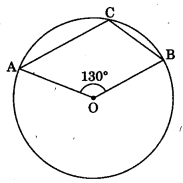CBSE Class 9 Circles MCQ New

## CBSE Class 9 Circles MCQ By Clarify Knowledge

CBSE Class 9 Circles MCQ New Pattern 2022

## CBSE Class 9 Circles MCQ Table

Question 1.
The radius of a circle is 13 cm and the length of one of its chords is 10 cm. The distance of the chord from the centre is
(a) 11.5 cm
(b) 12 cm
(c) 69−−√ cm

Question 2.
A chord is at a distance of 8 cm from the centre of a circle of radius 17 cm. The length of the chord is
(a) 25 cm
(b) 12.5 cm
(c) 30 cm

Question 3.
In the given figure, BOC is a diameter of a circle and AB = AC. Then, ∠ABC =(a) 30°
(b) 45°
(c) 60°

Question 4.
In the given figure, O is the centre of a circle. If ∠OAB = 40° and C is a point on the circle, then ∠ACB =(a) 40°
(b) 50°
(c) 80°

Question 5.
AB and CD are two equal chords of a circle with centre O such that ∠AOB = 80°, then ∠COD =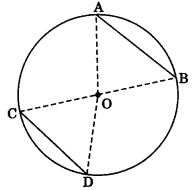(a) 100°
(b) 80°
(c) 120°

Question 6.
In the given figure, BOC is a diameter of a circle with centre O. If AB and CD are two chords such that AB || CD and AB = 10 cm, then CD =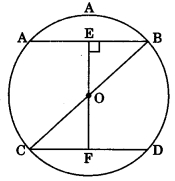(a) 5 cm
(b) 12.5 cm
(c) 15 cm

Question 7.
In the given figure, AB is a chord of a circle with centre O and AB is produced to C such that BC = OB. Also, CO is joined and produced to meet the circle in D. If ∠ACD = 25°, then ∠AOD =(a) 50°
(b) 75°
(c) 90°

Question 8.
In the given figure, AB is a chord of a circle with centre O and BOC is a diameter. If OD ⊥ AB such that OD = 6 cm, then AC =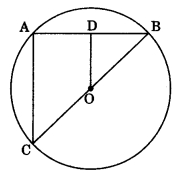(a) 9 cm
(b) 12 cm
(c) 15 cm

Question 9.
An equilateral triangle of side 9 cm is inscribed a circle. The radius of the circle is
(a) 3 cm
(b) 3√2 cm
(c) 3√3 cm

Question 10.
In the given figure, ΔABC and ΔDBC are inscribed in a circle such that ∠BAC = 60° and ∠DBC = 50°. then, ∠BCD =(a) 50°
(b) 60°
(c) 70°

Question 11.
In the given figure, O is the centre of a circle. If ∠AOB = =100° and ∠AOC = 90°, then ∠BAC =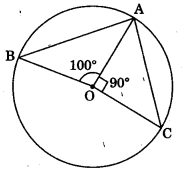(a) 85°
(b) 80°
(c) 90°

Question 12.
In the given figure, O is the centre of a circle and ∠AOC = 120°. Then, ∠BDC =(a) 60°
(b) 45°
(c) 30°

Question 13.
In the given figure, O is the centre of a circle in which ∠OAB = 20° and ∠OCB = 50°. Then, ∠AOC =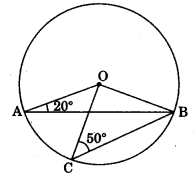(a) 50°
(b) 70°
(c) 20°

Question 14.
In the given figure, O is the centre of a circle in which ∠AOC = 100° side AB of quadrilateral OABC has been produced to D. Then, ∠CBD =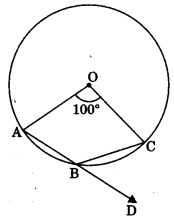(а) 50°
(b) 40°
(c) 25°

Question 15.
In the given figure, O is the centre of a circle and ∠OAB = 50°. Then, ∠BOD =(а) 130°
(b) 50°
(c) 100°

Question 16.
In the given figure, ABCD is the cyclic quadrilateral in which BC = CD and ∠CBD = 35°. Then, ∠BAD =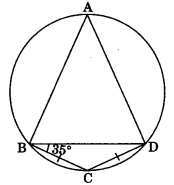(а) 65°
(b) 70°
(c) 110°

Question 17.
In figure, ΔABC is an equilateral triangle and ABDC is a quadrilateral then ∠BDC =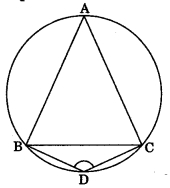(а) 90°
(b) 60°
(c) 120°

Question 18.
In figure, sides AB and AD of a quadrilateral ABCD are produced to E and F respectively. If ∠CBE = 100°, then find ∠CDF.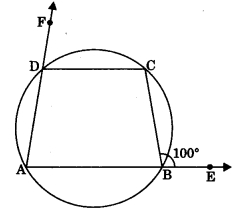(a) 100°
(b) 80°
(c) 130°

Question 19.
In figure, O is the centre of a circle and ∠AOB = 140°, then ∠ACB =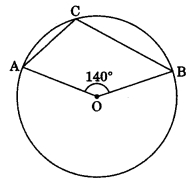(a) 70°
(b) 80°
(c) 110°